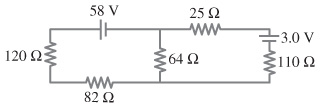# Problem: Calculate the currents in the below resistors of the figure.Part CCalculate the current in 64 ohm resistor.Express your answer to two significant figures and include the appropriate units.Part DCalculate the current in 110 ohm resistor.Express your answer to two significant figures and include the appropriate units.Part ECalculate the current in 82 ohm resistor.Express your answer to two significant figures and include the appropriate units.

###### FREE Expert Solution
89% (149 ratings)View Complete Written Solution
###### Problem DetailsCalculate the currents in the below resistors of the figure.

Part C

Calculate the current in 64 ohm resistor.

Express your answer to two significant figures and include the appropriate units.

Part D

Calculate the current in 110 ohm resistor.

Express your answer to two significant figures and include the appropriate units.

Part E

Calculate the current in 82 ohm resistor.

Express your answer to two significant figures and include the appropriate units.